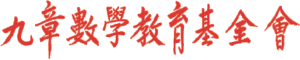首頁新聞區討論區檔案下載重要公告

 歷史公告

### 中學數學競賽

#### HOMC 2019

 主選單
 · 回首頁· 新聞區 · 討論區 · 檔案下載 · 網站連結 · 電子相薄 · 夥伴網站 · 精華文章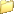討論區主頁10小學请教大家！ 限會員
 全部展開樹狀顯示 舊的在前 新的在前

Just popping in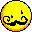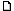请教大家！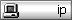我是北京的一名四年级小学生，首先向大家问好！请教高手及老师们：第十四届小学数学邀请赛的详解哪里有啊？团体的那道三角平方数的题，好难啊！2011-09-10 09:24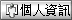Moderator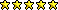Re: 请教大家！您才是大內高手, 由您的語氣中猜想您已經解出大部份的題目. 別說四年級學生, 在臺灣連六年級學生能正確解出一半的題目就算非常不錯的資優生了.原題目是:7. 已知888個連續正整數之和n + (n+1) + (n+2) + (n+3) + … + (n+886) + (n+887)是一個完全平方數。請問最小可能的正整數n值是什麼？n + (n+1) + (n+2) + … + (n+886) + (n+887) = 888n + = 4×111×(2n+887)。因其值為完全平方數，故知必存在正整數A使得2n+887=111×A×A。故知n的最小值與A的最小值同時發生，即A=3時，此時n=(999－887)÷2=56。_________________孫文先 敬上2011-09-10 13:04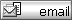Just popping inRe: 请教大家！非常感谢感谢孙老师！在此祝孙老师以及坛内朋友们中秋节阖家欢乐，身体健康！据我们老师讲，台湾的数学非常厉害，IMO金牌数量超过大陆！两岸的交流我仅仅知道有两岸四地精英赛，不知有没有机会参加？另外我想问的题目是Question4: 一个正整数如果是个三角形数且是完全平方数，则称为“保良数”.......请问比36大的下一个保良数是什么？谢谢老师！引文:孫文先 寫道:您才是大內高手, 由您的語氣中猜想您已經解出大部份的題目. 別說四年級學生, 在臺灣連六年級學生能正確解出一半的題目就算非常不錯的資優生了.原題目是:7. 已知888個連續正整數之和n + (n+1) + (n+2) + (n+3) + … + (n+886) + (n+887)是一個完全平方數。請問最小可能的正整數n值是什麼？n + (n+1) + (n+2) + … + (n+886) + (n+887) = 888n + = 4×111×(2n+887)。因其值為完全平方數，故知必存在正整數A使得2n+887=111×A×A。故知n的最小值與A的最小值同時發生，即A=3時，此時n=(999－887)÷2=56。2011-09-11 08:18ModeratorRe: 请教大家！4. 一個正整數如果是個三角形數且是個完全平方數，則稱它是個“保良數”。例如：36就是一個保良數，因為36 = 1+2+3+…+8（是個三角形數），且36 = 6 × 6 （是個完全平方數）。請問比36大的下一個保良數是什麼？註：一個三角形數為從1開始的若干個連續正整數之和，例如：1、3=1+2、6=1+2+3、10=1+2+3+4、…等等。一個完全平方數為一個正整數與自身的乘積，例如：1 = 1×1、4= 2 × 2、9 = 3 × 3、16 = 4 × 4、…等等。【解】由題意可知若n是保良數，則存在正整數k、h使得k(k+1)/2=h×h ，即 k(k+1)=2h×h。可假設h>6，且可由k與k+1互質知：i. h不可為 p^m的型態，其中m為正整數而p為質數，否則k=2而 p^2m，矛盾；ii. h不可為2p的型態，其中p為質數，否則k= 2×2×2=8而 k+1=p×p，即得p=3，故h=6，矛盾；若h=12= 2×2××3，則 k(k+1)=2^5×3^2，此時k無解，不合；若h=15=3×5，則 k(k+1)=2×3^2×5^2，此時k無解，不合；若h=18=2×3×3 ，則 k(k+1)=2^3×3^4，此時k無解，不合；若h=20= 2×2×5，則 k(k+1)=2^5×5^2，此時k無解，不合；若h=21=3×7，則 k(k+1)=2×3^2×7^2，此時k無解，不合；若h=24= 2×2×2×3，則 k(k+1)=2^7×3^2，此時k無解，不合；若h=28= 2×2×7，則 k(k+1)=2^5×7^2，此時k無解，不合；若h=30=2×3×5，則 k(k+1)=2^3×3^2×5^2，此時k無解，不合；若h=33=3×11，則 k(k+1)=2×3^2×11^2，此時k無解，不合；若h=35=5×7，則 k(k+1)=2×5^2×7^2，此時可取k=49、k+1=50。因此比36大的下一個保良數是 =1+2+3+…+49=1225。答：1225_________________孫文先 敬上2011-09-11 14:50ModeratorRe: 请教大家！Dear 孫老師:我找出通解方法是用豆子表示出「一三角形數等於一正方形數」和「一三角形數乘2等於一正方形數」的關聯結果是遞回式若a^2=1+2+......+b則(3a+2b+1)^2=1+2+......+(4a+3b+1)可用豆子證明這樣可造出所有可能值 林鼎鈞敬上_________________孫文先 敬上2011-09-14 20:27全部展開樹狀顯示 舊的在前 新的在前

 選擇討論區   九章數學基金會 ---------------- 讀書討論區 2012國際數學競賽專區   數學討論區 ---------------- 學習討論區 數學玩具討論區 小學 國中 高中 教學 其他 家長   九章數學教育基金會各項活動的感想與建議 ---------------- 參加小學數學競賽的感想與建議 參加中學數學競賽的感想與建議 參加九章數學俱樂部的感想與建議 參加奧林匹克訓培營的感想與建議 參加加拿大英文及數學夏令營的感想與建議 其它九章數學教育基金會活動的感想與建議   站內事務區 ---------------- 本站使用協助與建議區   九章數學愛好者聯誼會員專區 ---------------- 九章數學愛好者聯誼會員專區九章數學出版社、九章數學基金會版權所有本網頁各鍊結標題及鍊結內容歸原權利人所有Copyright 2000 ~2004九章數學出版社、九章數學基金會本網站內所有文字及資料版權均屬九章所有，未經書面同意之商業用途必究This web site was made with XOOPS, a web portal system written in PHP. XOOPS is a free software released under the GNU/GPL license.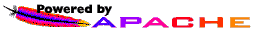Powered by XOOPS 1.3.10 © 2002 The XOOPS Project